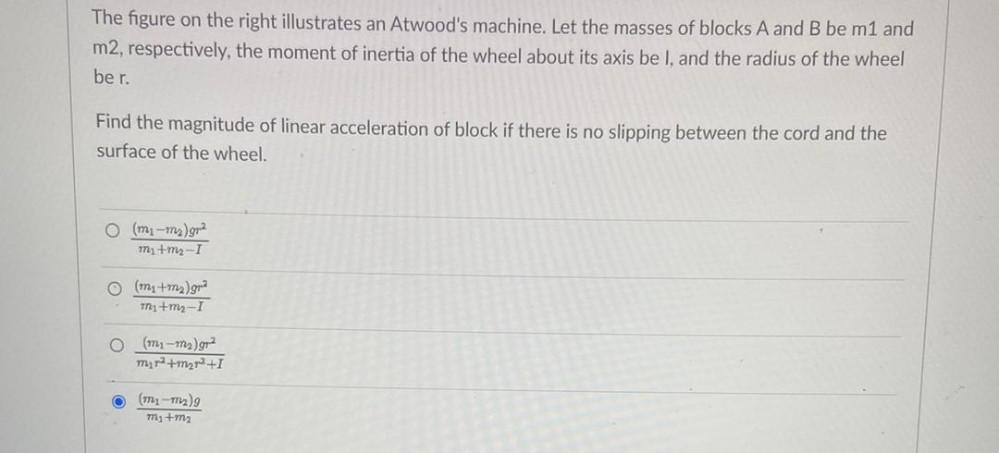Question:

# The figure on the right illustrates an Atwood's machine. Let the masses of blocks A and B be m1 and m2, respectively, the momentThe figure on the right illustrates an Atwood's machine. Let the masses of blocks A and B be m1 and m2, respectively, the moment of inertia of the wheel about its axis be I, and the radius of the wheel ber. Find the magnitude of linear acceleration of block if there is no slipping between the cord and the surface of the wheel. (mi-my)gra mitm-I (mitm)gma Mi+m2-I (mı-my)g2 mira+mzr2+1 (1721 m2) m+mz